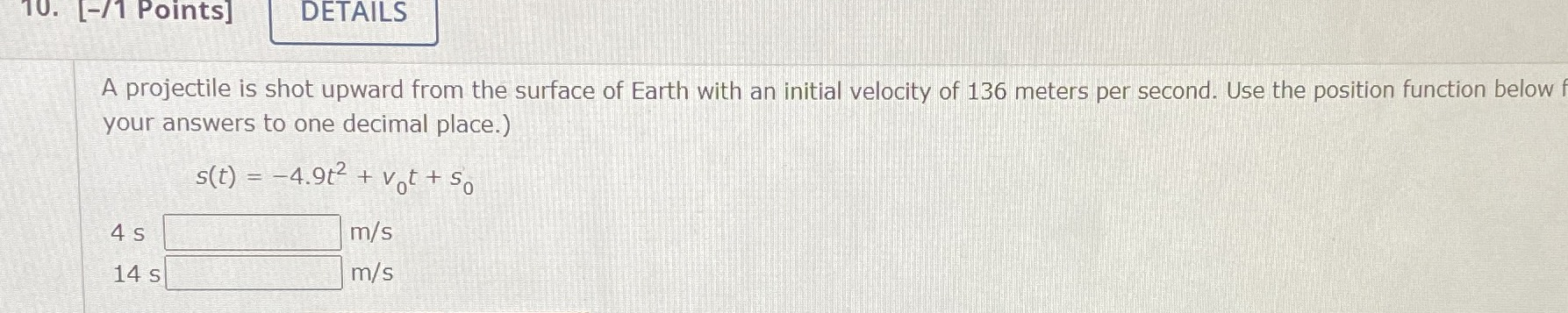### ¿Todavía tienes preguntas de matemáticas?

Pregunte a nuestros tutores expertos
Algebra
Pregunta10. [-11 Points] DETAILS A projectile is shot upward from the surface of Earth with an initial velocity of $$136$$ meters per second. Use the position function below f your answers to one decimal place.)

$$s ( t ) = - 4.9 t ^ { 2 } + v _ { 0 } t + s _ { 0 }$$

$$116.4\\67.4$$